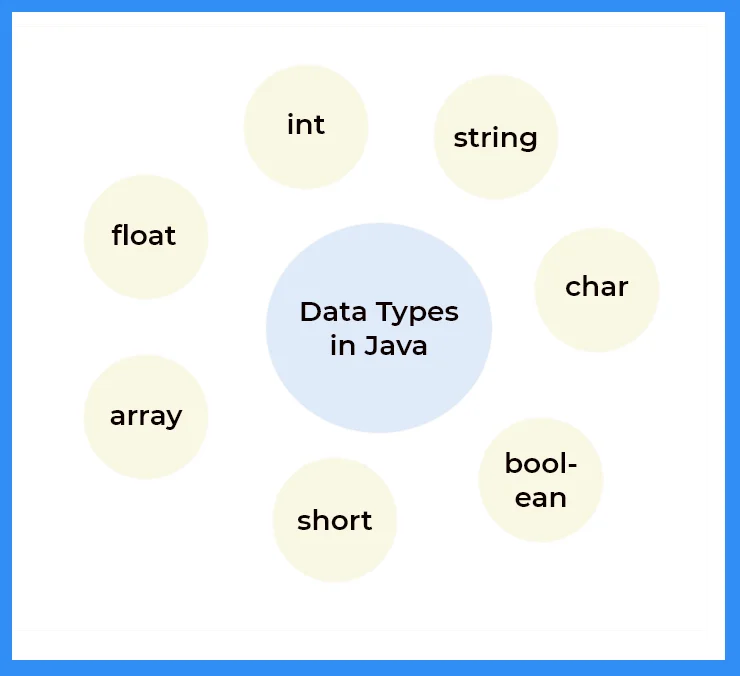# Data Types in Java

## Data Types In Java

Here, on this page we will discuss about  all the Data Types present in Java Programming Language. Java is a statically-typed programming language, which means that all variables must have a declared data type before they can be used.  There are mainly two types of Data Type:Data TypeDefault ValueDefault Size
Boolean False1 bit
Char‘\u0000’.2 Byte
Byte01 byte
Short02 byte
Int04 byte
Long0L8 byte
Float0.0f4 byte
Double0.0d8 byte

## Primitive Data Type

Predefined data types that are supported by a programming language and cannot be broken down into further simpler types are known as Primitive data types.

We have eight Primitive data types namely;-

• boolean
• char
• byte
• short
• int
• long
• float
• double

### Related Banners

###### 1. Boolean
• The boolean data type allows the programmer to store only two values- True or False.
• Hence it represents only one bit of information but the size of this data type is implementation dependent.
• It is often used as simple flag that is used to determine a True / False situation.

Run

```public class Main
{
public static void main(String[] args) {
boolean a = true;
if (a == true)
System.out.println("Hey There Prepster");
}
}```

`Output :Hey There Prepster`
###### 2. Char
• The char data type is typically used to store a single Unicode character, and it is often represented as 16 bits (2 bytes) in many programming languages.
• Its value range spans from ‘\u0000’ (which is equivalent to 0) to ‘\uffff’ (equivalent to 65,535), inclusive.
• This data type is primarily employed for character storage and manipulation in various programming contexts.
Run
```public class CharExample {
public static void main(String[] args) {
char myChar = 'A';
System.out.println("The value of myChar is: " + myChar);
}
}
```
```Output :The value of myChar is: A
```
###### 3. Byte
• The Byte data type is an 8-bit signed two’s complement integer.
• This can be used instead of int or other integer types to save memory when we are certain that the value will be within -128 and 127, since byte is 4 times smaller than the int type.
• A special feature of this data type is that it is cyclic in nature.
Run
```class Main {
public static void main(String[] args) {
byte num;

num = 126;

num++;

System.out.println(num);

num++;

System.out.println(num);

}
}```
`Output :127-128`
###### 4. Short
• The short data type is a 16-bit signed two’s complement integer.
• Its value-range lies between -32,768 to 32,767 (inclusive). Its minimum value is -32,768 and maximum value is 32,767. Its default value is 0.
• The short data type can also be used to save memory in large arrays , just like byte data type. A short data type is 2 times smaller than an integer.
Run
```public class Main
{
public static void main(String[] args) {
short num = 10000;
System.out.println(num);
}
}```
`Output :10000`
###### 5. Int
• The int data type is the preferred data type when we create variables with a numeric value.
Run
```public class Main {
public static void main(String[] args) {
int myInt = 42; // Declare an int variable and assign a value to it
System.out.println("The value of myInt is: " + myInt); // Print the value of the int variable
}
}

}```
```Output :
The value of myInt is: 42
```
###### 6. Long
• The long data type is a 64-bit integer that employs a two’s complement representation.
• Its value range extends from -9,223,372,036,854,775,808 (which is equivalent to -2^63) to 9,223,372,036,854,775,807 (which is equivalent to 2^63 – 1), inclusive.
• The minimum value it can hold is -9,223,372,036,854,775,808, while the maximum value is 9,223,372,036,854,775,807.
• By default, a long variable is initialized to 0. This data type is utilized when a wider range of values is required compared to the int data type
Run
```public class LongExample {
public static void main(String[] args) {
// Define two long integers and calculate their sum
long num1 = 123456789012345L;
long num2 = 987654321098765L;
long sum = num1 + num2;

// Display the result
System.out.println("Sum of " + num1 + " and " + num2 + " is: " + sum);
}
}
```
```Output :
Sum of 123456789012345 and 987654321098765 is: 1111111111111110

```
###### 7. Float
• The float data type is a 32-bit IEEE 754 single-precision floating-point representation.
• It does not have a limited value range, which means it can represent a wide range of values, including very large and very small numbers.
• It is advisable to use float instead of double when conserving memory is essential, especially in situations involving large arrays of floating-point numbers.
Run
```float myFloat = 3.14f;
System.out.println(myFloat);
```
```Output :
3.14
```
###### 8. Double
• The double data type is a 64-bit IEEE 754 double-precision floating-point format.
• It possesses an extensive value range, accommodating a wide variety of numeric values, including those of substantial magnitude or extreme precision. Similar to the float data type, double is commonly used for decimal values.
• However, it’s crucial to note that for applications requiring high precision, such as currency calculations, it is not recommended to use the double data type due to its inherent limitations.
Run
```public class DoubleExample {
public static void main(String[] args) {
// Define two double numbers and calculate their sum
double num1 = 3.14159265359;
double num2 = 2.71828182846;
double sum = num1 + num2;

// Display the result
System.out.println("Sum of " + num1 + " and " + num2 + " is: " + sum);
}
}

```
```Output :
Sum of 3.14159265359 and 2.71828182846 is: 5.85987448205

```

Get PrepInsta Prime & get Access to all 200+ courses offered by PrepInsta in One Subscription

## Get over 200+ course One Subscription

Courses like AI/ML, Cloud Computing, Ethical Hacking, C, C++, Java, Python, DSA (All Languages), Competitive Coding (All Languages), TCS, Infosys, Wipro, Amazon, DBMS, SQL and others